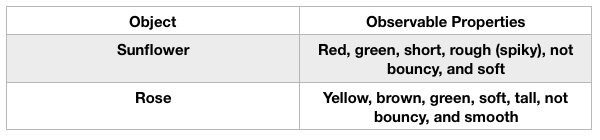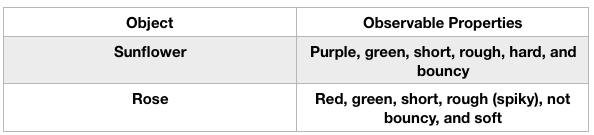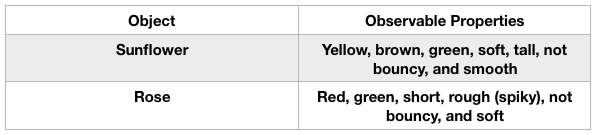# 2nd Grade Science : Physical Science

## Example Questions

← Previous 1 3 4 5 6 7 8 9 10 11

### Example Question #1 : Physical Science

Select the tool you would use to answer the question provided.

How hot is the hot chocolate?

Measuring cup

Ruler

Thermometer

Scale

Thermometer

Explanation:

Let's take a look at the tools given in the answer choices:

A thermometer is used to measure the temperature of something, or to determine how hot or cold something is.

A scale is used to determine the weight of an object.

A measuring cup is used to determine the amount of something. Measuring cups are often used in baking. For example, if a recipe called for two cups of sugar, you would use a measuring cup to measure out two cups of sugar.

A ruler is used to measure the length of something.

Based on the question, we are trying to determine how hot the hot chocolate is, so we would use a thermometer.

### Example Question #7 : 2nd Grade Science

Select the tool you would use to answer the question provided.

How heavy is the book?

Scale

Measuring cup

Thermometer

Ruler

Scale

Explanation:

Let's take a look at the tools given in the answer choices:

A thermometer is used to measure the temperature of something, or to determine how hot or cold something is.

scale is used to determine the weight of an object, or to determine how heavy or light something is.

measuring cup is used to determine the amount of something. Measuring cups are often used in baking. For example, if a recipe called for two cups of sugar, you would use a measuring cup to measure out two cups of sugar.

ruler is used to measure the length of something.

Based on the question, we are trying to determine how heavy the book is, so we would use a scale.

### Example Question #1 : Physical Science

Select the tool you would use to answer the question provided.

What is the temperature outside?

Thermometer

Scale

Ruler

Measuring cup

Thermometer

Explanation:

Let's take a look at the tools given in the answer choices:

A thermometer is used to measure the temperature of something, or to determine how hot or cold something is.

scale is used to determine the weight of an object.

measuring cup is used to determine the amount of something. Measuring cups are often used in baking. For example, if a recipe called for two cups of sugar, you would use a measuring cup to measure out two cups of sugar.

ruler is used to measure the length of something.

Based on the question, we are trying to determine the temperature, so we would use a thermometer.

### Example Question #2 : Physical Science

Select the tool you would use to answer the question provided.

How long is the desk?

Thermometer

Scale

Ruler

Measuring cup

Ruler

Explanation:

Let's take a look at the tools given in the answer choices:

A thermometer is used to measure the temperature of something, or to determine how hot or cold something is.

scale is used to determine the weight of an object.

measuring cup is used to determine the amount of something. Measuring cups are often used in baking. For example, if a recipe called for two cups of sugar, you would use a measuring cup to measure out two cups of sugar.

ruler is used to measure the length of something.

Based on the question, we are trying to determine how long the desk is, so we would use a ruler.

### Example Question #1 : Investigate Materials And Observable Properties

What are the observable properties that we can use to describe materials?

Color, texture, hardness, and flexibility

Length, width, and height

Color, width, texture, and volume

Temperature, weight, density, and volume

Color, texture, hardness, and flexibility

Explanation:

Observable properties are characteristics or things about materials or objects that we can describe using our five senses. Color, texture, hardness, and flexibility are all things we can determine with our senses.

### Example Question #1 : Investigate Materials And Observable Properties

What does the word observable mean?

To be served

To go unnoticed

To be noticed

To serve someone

To be noticed

Explanation:

The term observable means to be noticed or perceived. If something is noticeable, I can touch it, taste it, see it, hear it, or feel it. We can observe the properties of a material and use it to determine what it is or how to classify it.

### Example Question #3 : Investigate Materials And Observable Properties

What is another term for materials? Example: I can observe the properties of different materials in the classroom.

Matter

Desks

Blankets

Chairs

Matter

Explanation:

Another term for materials is matter. Matter is what things are made of. All materials, objects, and living things are made of matter.

### Example Question #1 : Classification Of Materials

Which object matches the list of observable properties below?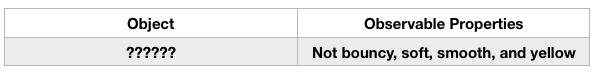A ping pong ball

An apple

A banana

A banana

Explanation:

The observable properties listed give us a clue to what the mystery object is. We know the object needs to be yellow, soft, smooth, and it should not bounce. An apple is typically not yellow, a ping pong ball IS bouncy, and basketballs are generally bouncy and orange, so they are not a match. That leaves a banana which meets all the observable properties.

### Example Question #5 : Investigate Materials And Observable Properties

What is Janice describing with the observable properties data table below?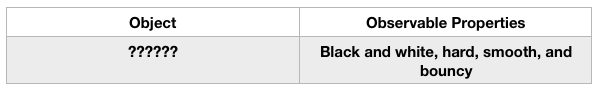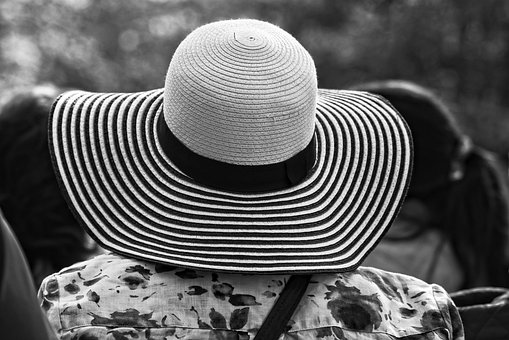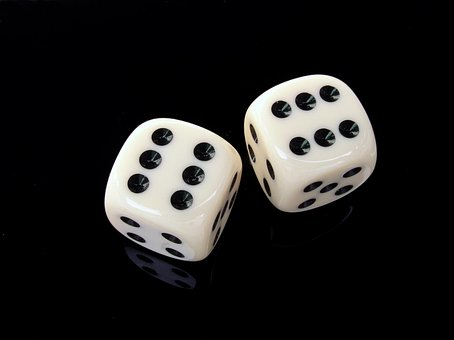Explanation:

The object that Janice is describing is a die or pair of dice. They are bouncy, hard, smooth, and black and white. The other choices are black and white but don't meet the other observable properties.

### Example Question #1 : Physical Science

JR has a sunflower and a rose and wants to investigate their observable properties. Which data table matches the properties of the two objects?